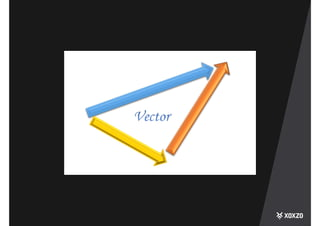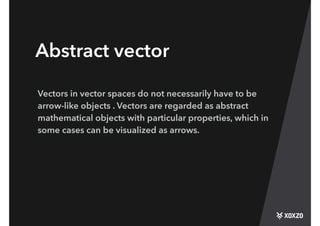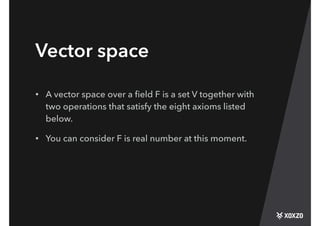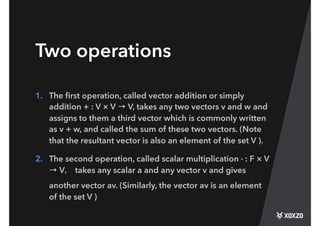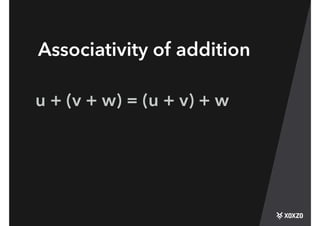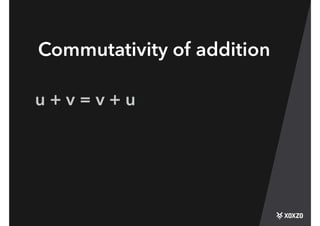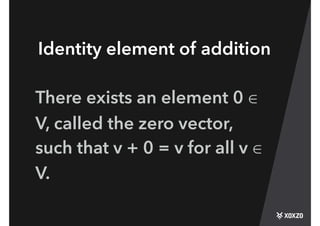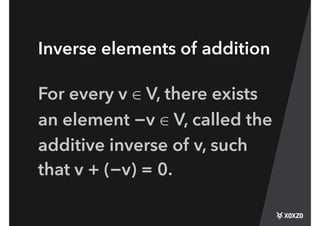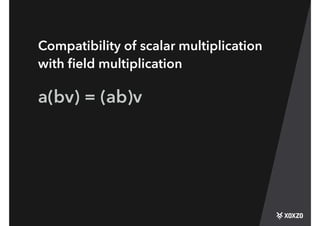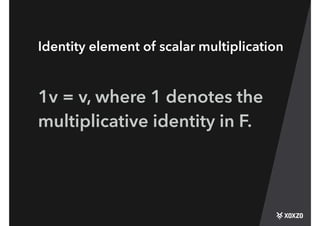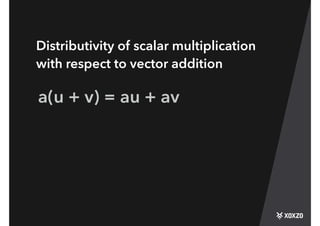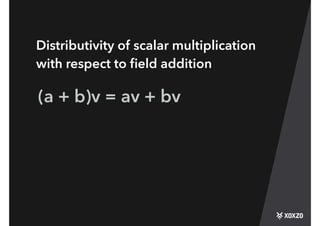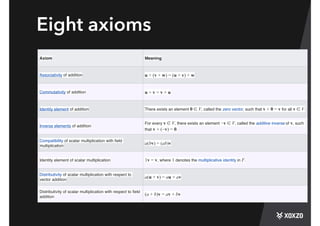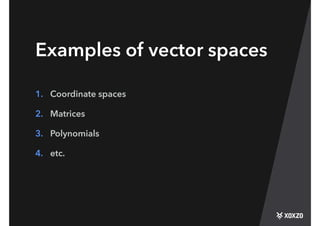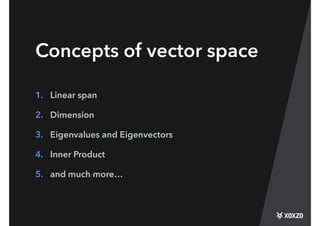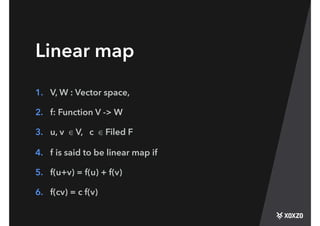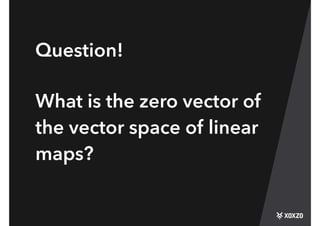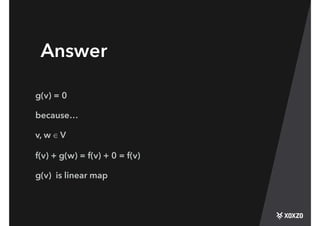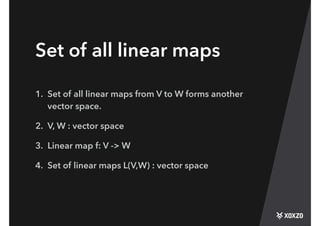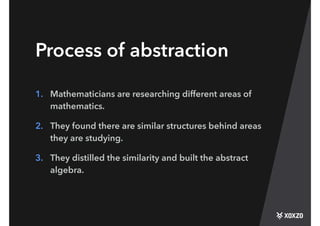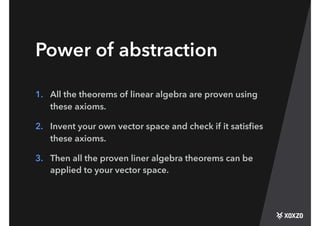1 de 24

### Linear algebra power of abstraction - LearnDay@Xoxzo #5

1. Linear Algebra The power of abstraction Akira Nonaka
2. Abstract vector Vectors in vector spaces do not necessarily have to be arrow-like objects . Vectors are regarded as abstract mathematical objects with particular properties, which in some cases can be visualized as arrows.
3. Vector space • A vector space over a ﬁeld F is a set V together with two operations that satisfy the eight axioms listed below. • You can consider F is real number at this moment.
4. Two operations 1. The ﬁrst operation, called vector addition or simply addition + : V × V → V, takes any two vectors v and w and assigns to them a third vector which is commonly written as v + w, and called the sum of these two vectors. (Note that the resultant vector is also an element of the set V ). 2. The second operation, called scalar multiplication · : F × V → V， takes any scalar a and any vector v and gives another vector av. (Similarly, the vector av is an element of the set V )
5. Axioms
6. Associativity of addition u + (v + w) = (u + v) + w
7. Commutativity of addition u + v = v + u
8. Identity element of addition There exists an element 0 ∈ V, called the zero vector, such that v + 0 = v for all v ∈ V.
9. Inverse elements of addition For every v ∈ V, there exists an element −v ∈ V, called the additive inverse of v, such that v + (−v) = 0.
10. Compatibility of scalar multiplication with ﬁeld multiplication a(bv) = (ab)v
11. Identity element of scalar multiplication 1v = v, where 1 denotes the multiplicative identity in F.
12. a(u + v) = au + av Distributivity of scalar multiplication with respect to vector addition
13. (a + b)v = av + bv Distributivity of scalar multiplication with respect to ﬁeld addition
14. Eight axioms Axiom Meaning Associativity of addition u + (v + w) = (u + v) + w Commutativity of addition u + v = v + u Identity element of addition There exists an element 0 ∈ V, called the zero vector, such that v + 0 = v for all v ∈ V. Inverse elements of addition For every v ∈ V, there exists an element −v ∈ V, called the additive inverse of v, such that v + (−v) = 0. Compatibility of scalar multiplication with ﬁeld multiplication a(bv) = (ab)v Identity element of scalar multiplication 1v = v, where 1 denotes the multiplicative identity in F. Distributivity of scalar multiplication with respect to vector addition a(u + v) = au + av Distributivity of scalar multiplication with respect to ﬁeld addition (a + b)v = av + bv
15. Examples of vector spaces 1. Coordinate spaces 2. Matrices 3. Polynomials 4. etc.
16. Concepts of vector space 1. Linear span 2. Dimension 3. Eigenvalues and Eigenvectors 4. Inner Product 5. and much more…
17. Linear map 1. V, W : Vector space, 2. f: Function V -> W 3. u, v ∈ V, c ∈ Filed F 4. f is said to be linear map if 5. f(u+v) = f(u) + f(v) 6. f(cv) = c f(v)
18. Question! What is the zero vector of the vector space of linear maps?
19. Answer g(v) = 0 because… v, w ∈ V f(v) + g(w) = f(v) + 0 = f(v) g(v) is linear map
20. Set of all linear maps 1. Set of all linear maps from V to W forms another vector space. 2. V, W : vector space 3. Linear map f: V -> W 4. Set of linear maps L(V,W) : vector space
21. Process of abstraction 1. Mathematicians are researching different areas of mathematics. 2. They found there are similar structures behind areas they are studying. 3. They distilled the similarity and built the abstract algebra.
22. Power of abstraction 1. All the theorems of linear algebra are proven using these axioms. 2. Invent your own vector space and check if it satisﬁes these axioms. 3. Then all the proven liner algebra theorems can be applied to your vector space.
23. Reference 1. https://en.wikipedia.org/wiki/Vector_space 2. https://en.wikipedia.org/wiki/Linear_map 3. https://youtu.be/ozwodzD5bJM